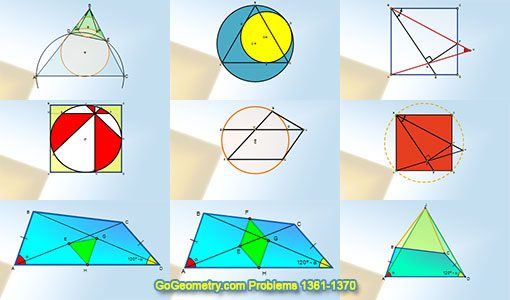# Geometry Problems 1361-1370. Level: High School, CollegeGeometry Problems 1361 - 1370Geometry Problem 1361. Triangle, Two Nine-Point Circles, Feuerbach's Circle, Euler's Circle, Congruent Angles, Sketch, iPad Apps. Geometry Problem 1362. Equilateral Triangle Inscribed in a Circle, Lunula, Ratio of Areas, Tangent, Sketch, iPad Apps. Geometry Problem 1363. Square, Angle Bisector, Perpendicular, 45 Degrees, Angle, Sketch, iPad Apps. Geometry Problem 1364. Square, Inscribed Circle, Area, Angle Bisector, Perpendicular, 45 Degrees, Sketch, iPad Apps. Geometry Problem 1365. Circle, Tangent, Secant, Chord, Parallel, Midpoint, Sketch, iPad Apps. Geometry Problem 1366. Square, Angle Bisector, Perpendicular, Concyclic Points, Circle, Sketch, iPad Apps.

Home | Sitemap | Geometry | Problems | Visual Index | 10 ProblemsEmail | Post a comment | by Antonio Gutierrez
Last updated: Aug 18, 2018